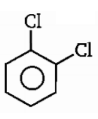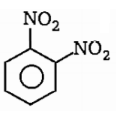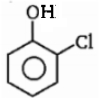Incorrect statement regarding ${\mathrm{BF}}_{2}{\mathrm{NH}}_{2}$ molecule is :

(a) FBF bond angle $<120%$                      (b) HNH bond angle $>109°28\text{'}$

(c) Exhibits intermolecular H-bond             (d) Hybridization of N-atom is ${\mathrm{sp}}^{3}$

Concept Questions :-

Hybridisation
High Yielding Test Series + Question Bank - NEET 2020

Difficulty Level:

In which of the following molecule $\mu$ (observed) is found to be greater than $\mu$ (theoretical)

1. ${\mathrm{CHCl}}_{3}$
2.3.4.Concept Questions :-

Polarity
High Yielding Test Series + Question Bank - NEET 2020

Difficulty Level:

The bond having minimum bond energy is :

(a) $\mathrm{C}—\mathrm{C}$                              (b) $\mathrm{O}—\mathrm{O}$

(c) $\mathrm{S}—\mathrm{S}$                               (d) $\mathrm{P}—\mathrm{P}$

High Yielding Test Series + Question Bank - NEET 2020

Difficulty Level:

Which gas should not be collected over water because of its high solubility in water?

(a) ${H}_{2}$

(b) ${N}_{2}$

(c) $C{H}_{4}$

(d) HCl

High Yielding Test Series + Question Bank - NEET 2020

Difficulty Level:

The correct increasing order of extent of hydrolysis is :

(a) ${\mathrm{CCl}}_{4}<{\mathrm{MgCl}}_{2}<{\mathrm{AlCl}}_{3}<{\mathrm{SiCl}}_{4}<{\mathrm{PCl}}_{5}$           (b) ${\mathrm{CCl}}_{4}<{\mathrm{AlCl}}_{3}<{\mathrm{MgCl}}_{2}<{\mathrm{PCl}}_{5}<{\mathrm{SiCl}}_{4}$

(c) ${\mathrm{CCl}}_{4}<{\mathrm{SiCl}}_{4}<{\mathrm{PCl}}_{5}<{\mathrm{AlCl}}_{3}<{\mathrm{mgCl}}_{2}$            (d) ${\mathrm{CCl}}_{4}<{\mathrm{PCl}}_{5}<{\mathrm{SiCl}}_{4}<{\mathrm{AlCl}}_{3}<{\mathrm{MgCl}}_{2}$

Concept Questions :-

Lattice/Hydration Energy
High Yielding Test Series + Question Bank - NEET 2020

Difficulty Level:

Low melting point is expected for soild:

(a) Ionic soild

(b) metallic solid

(c) molecular soild

(d) covalent soild

Concept Questions :-

Lattice/Hydration Energy
High Yielding Test Series + Question Bank - NEET 2020

Difficulty Level:

Inorganic benzene reacts with HCl to form a compound ${\mathrm{B}}_{3}{\mathrm{N}}_{3}{\mathrm{H}}_{9}{\mathrm{Cl}}_{3}$. The protonation occurs at:

(a) B-atom

(b) N-atom

(c) First at B-atom then rearrangers into N-atom

(d) First N-atom then rearrangers into B-atom

Concept Questions :-

Hybridisation
High Yielding Test Series + Question Bank - NEET 2020

Difficulty Level:

Given the correct order of initials or F for the following statements. Use T if staement is true and F if it is false:

(i)is non-polar  is polar molecule

(ii) ${\mathrm{CH}}_{3}\stackrel{^}{\mathrm{P}}{\mathrm{CH}}_{3}$ bond angles are equal in  molecule

(iii) ${\mathrm{PF}}_{3}$ will be more soluble in polar solvent than ${\mathrm{SiF}}_{4}$

(a) TTF                (b) FFT                     (c) FFF                           (d) FTT

Concept Questions :-

Polarity
High Yielding Test Series + Question Bank - NEET 2020

Difficulty Level:

Which substance has the strongest London dispersion forces?

(a) $Si{H}_{4}$

(b) $C{H}_{4}$

(c) $Sn{H}_{4}$

(d) $Ge{H}_{4}$

Concept Questions :-

Types of Bonding
High Yielding Test Series + Question Bank - NEET 2020

Difficulty Level:

Which of the following compounds has the lowest boiling point?

(a) HF

(b) HCl

(c) HBr

(d) HI

Concept Questions :-

Hydrogen Bonding